• 主要介绍了Python NumPy库安装使用笔记,本文讲解了NumPy的安装和基础使用,并对每一句代码都做了详细解释,需要的朋友可以参考下
• 主要介绍了Python Numpy库安装与基本操作,简单介绍了Numpy库的基本功能、并结合实例形式分析了基于Numpy库的数组与矩阵相关操作技巧,需要的朋友可以参考下
• [root@Singapore numpy]# wget https://pypi.python.org/packages/ee/66/7c2690141c520db08b6a6f852fa768f421b0b50683b7bbcd88ef51f33170/numpy-1.14.0.zip[root@Singapore numpy]# md5sum numpy-1.14.0.zipc12d4bf3...
[root@Singapore numpy]# wget https://pypi.python.org/packages/ee/66/7c2690141c520db08b6a6f852fa768f421b0b50683b7bbcd88ef51f33170/numpy-1.14.0.zip
[root@Singapore numpy]# md5sum numpy-1.14.0.zip
[root@Singapore numpy]# unzip numpy-1.14.0.zip
[root@Singapore numpy]# cd numpy-1.14.0
[root@Singapore numpy-1.14.0]# cat INSTALL.rst.txt #安装说明
[root@Singapore numpy-1.14.0]# python3 setup.py build install --prefix /root/python/numpy #注意安装路径
[root@Singapore numpy-1.14.0]# echo "export PYTHONPATH=/root/python/numpy/lib/python3.6/site-packages" >> ~/.bashrc #注意安装路径
[root@Singapore numpy-1.14.0]# . ~/.bashrc
[root@Singapore numpy-1.14.0]# echo \$?
0
[root@Singapore numpy-1.14.0]#
写一个线性回归 试一试[root@Singapore work.dir]# cat SimpleLineRegression.py
#!/usr/bin/python3
import numpy as np
def fitSLR(x,y):
n = len(x)
dinominator = 0
numerator = 0
for i in range(0, n):
numerator += (x[i] - np.mean(x)) * (y[i] - np.mean(y))
dinominator +=(x[i] - np.mean(x)) ** 2
print ("numerator:", numerator)
print ("dinominator", dinominator)
b1 = numerator/float(dinominator)
b0 = np.mean(y)/float(np.mean(x))
return b0, b1
def predict(x, b0, b1):
return b0 + x*b1
x = [1,3,2,1,3]
y = [14,24,18,17,27]
b0, b1 = fitSLR(x,y)
print ("intercept:", b0, " slope:", b1)
x_test = 6
y_test = predict(6, b0, b1)
print("y_test", y_test)
[root@Singapore work.dir]# ./SimpleLineRegression.py
numerator: 20.0
dinominator 4.0
intercept: 10.0 slope: 5.0
y_test 40.0
[root@Singapore work.dir]#
展开全文• ## PythonNumpy库教程

万次阅读 多人点赞 2018-06-02 15:10:47
本章节主要介绍的是python的一个常用库numpy，通过本章教程希望能够掌握numpy库一些常用的方法。 简介 NumPy 是一个 Python 的第三方库，代表 “Numeric Python”，主要用于数学/科学计算。 它是一个由多维数组...
本章节主要介绍的是python的一个常用库numpy，通过本章教程希望能够掌握numpy库一些常用的方法。
简介
NumPy 是一个 Python 的第三方库，代表 “Numeric Python”，主要用于数学/科学计算。 它是一个由多维数组对象和用于处理数组的例程集合组成的库。
使用 Numpy 我们可以轻松进行如下等计算：
数组的算数和逻辑运算。傅立叶变换和用于图形操作的例程。与线性代数有关的操作。 NumPy 拥有线性代数和随机数生成的内置函数。
安装
推荐使用pip命令进行安装或者下载 Anaconda (一个python集成环境应用，内置numpy等常用库)
pip install numpy
检查
我们可以引入numpy，如果未报错说明已经正常安装
import numpy as np
开始
这里介绍一个概念：NumPy - Ndarray 对象，Ndarray对象是numpy里面最重要的一个 N维数组 对象。
调用numpy.array方法可以从任何暴露数组接口的对象，或从返回数组的任何方法创建一个ndarray类型的对象。
numpy.array(object, dtype = None, copy = True, order = None, subok = False, ndmin = 0)
语法：
参数描述object任何暴露数组接口方法的对象都会返回一个数组或任何(嵌套)序列。dtype数组的所需数据类型，可选。copy可选，默认为true，对象是否被复制。orderC(按行)、F(按列)或A(任意，默认)。subok默认情况下，返回的数组被强制为基类数组。 如果为true，则返回子类。ndimin指定返回数组的最小维数。
我们先看几个简单的栗子帮助我们理解：
使用numpy创建一维数组：
import numpy as np
list = [1,2,3,4]
oneArray = np.array(list)

print(list)     #[1, 2, 3, 4]  这是python的列表对象
print(oneArray) #[1 2 3 4]     这是一个一维数组

使用numpy创建二维数组：
import numpy as np
twoArray = np.array([[1,2],[3,4],[5,6]])

print(twoArray) #[[1 2] [3 4] [5 6]]  这是一个二维数组
数据类型：
了解了一些概念之后我们看一下numpy的数据类型：
类型描述bool存储为一个字节的布尔值(True或False)。int默认整数，通常为int32或int64。int8字节（-128 ~ 127）。int16整数（-32768 ~ 32767）。int32整数（-2 * 31 ~ 2 * 31 - 1）。int64整数（-2 * 63 ~ 2 * 63 - 1）。uint8无符号整数（0 ~ 255）。uint16无符号整数（0 ~ 65535）。uint32无符号整数（0 ~ 2 ** 32 - 1）。uint64无符号整数（0 ~ 2 ** 64 - 1）。float16半精度浮点，符号位，5 位指数，10 位尾数。float32单精度浮点，符号位，8 位指数，23 位尾数。float64或float双精度浮点，符号位，11 位指数，52 位尾数。complex64复数，由两个 32 位浮点表示（实部和虚部）。complex128或complex复数，由两个 64 位浮点表示（实部和虚部）。
NumPy 数字类型是dtype(数据类型)对象的实例，每个对象具有唯一的特征。
数据类型对象 (dtype)：
数据类型对象描述了对应于数组的固定内存块的解释，取决于以下方面：
数据类型(整数、浮点或者 Python 对象)数据大小字节序(小端或大端)在结构化类型的情况下，字段的名称，每个字段的数据类型，和每个字段占用的内存块部分。如果数据类型是子序列，它的形状和数据类型。
dtype构造语法：
numpy.dtype(object, align, copy)
参数描述Object存储为一个字节的布尔值(True或False)。Align如果为true，则向字段添加间隔，使其类似 C 的结构体。Copy生成dtype对象的新副本，如果为flase，结果是内建数据类型对象的引用。
示例：
import numpy as np

#int 32
dt = np.dtype(np.int32)
print(dt)

#int8，int16，int32，int64 可替换为等价的字符串 'i1'，'i2'，'i4'，以及其他。

dt4 = np.dtype('i4')
print(dt4) # int32

dt8 = np.dtype('i8')
print(dt8) # int64

# 使用端记号

dt11 = np.dtype('>i4')
print dt11 # >i4

# 创建结构化数据类型。

dts = np.dtype([('age',np.int8)])
print dts # [('age', 'i1')]

# 以下示例定义名为 student 的结构化数据类型，其中包含字符串字段name，整数字段age和浮点字段marks。 此dtype应用于ndarray对象

student = np.dtype([('name','S20'),  ('age',  'i1'),  ('marks',  'f4')])
print student

#[('name', 'S20'), ('age', 'i1'), ('marks', '<f4')])
dtype数组属性：
在了解了dtype数组的构造方法后，我们来了解dtype的数组属性。
方法描述ndarray.shape返回一个包含数组维度的元组，它也可以用于调整数组大小ndarray.reshape用来调整数组大小ndarray.ndim返回数组的维数。numpy.itemsize返回数组中每个元素的字节单位长度。
# ndarray.shape 返回一个包含数组维度的元组，它也可以用于调整数组大小

import numpy as np

a = np.array([[1,2,3],[4,5,6]])
print a.shape #(2,3) 表示这是一个二行三列的数组

----------
#改变数组的大小
b = a
b.shape =  (3,2)
print(b) # b成为了一个三行两列的新数组

# [
#  [1 2]
#  [3 4]
#  [5 6]
# ]

----------
# 直接使用reshape方法进行转化

c = a.reshape(3,2)
print(c)

# [
#  [1 2]
#  [3 4]
#  [5 6]
# ]

----------
# ndarray.ndim 返回数组的维数

d = np.array([1,2,3,4,5])
e = np.array([[1,2,3,4,5],[6,7,8,9,10]])

print(d.ndim) # 1
print(e.ndim) # 2

----------
# numpy.itemsize

f = np.array([1,2,3,4,5], dtype = np.int8)
print(f.itemsize) # 1 np.int8(一个字节)

g = np.array([1,2,3,4,5], dtype = np.float32)
print(g.itemsize) # 4 np.float32(四个字节)

#创建数组
使用numpy创建数组有多种形式，我们一一来了解一下
numpy.empty # 创建指定形状和dtype的未初始化数组 numpy.zeros # 返回特定大小，以 0 填充的新数组 numpy.ones # 返回特定大小，以 1 填充的新数组
这三种方法有相同的参数列表：
参数描述shape空数组的形状，整数或整数元组dtype所需的输出数组类型，可选order‘C’为按行的 C 风格数组，’F’为按列的 Fortran 风格数组
以下是这三种方法的Demo：
import numpy as np

# 使用 numpy.empty

x = np.empty([2,2], dtype = int)
print(x)

#由于我们未给定初始化值，所以初始化出来的值是随机int8类型

# [
#   [ 1152921504606846976 -8070441754919082034]
#   [ 4333764610           562954288073672]
# ]

----------
# 使用 numpy.zeros

y = np.zeros(, dtype = int)
print(y)

#我们使用zeros方法创建了4个0元素
# [0 0 0 0]

----------
# 使用 numpy.ones

z = np.ones(, dtype = int)
print(z)

#我们使用ones方法创建了3个1元素
# [1 1 1]
从已有的数据创建数组
numpy.asarray # 类似于numpy.array numpy.fromiter # 此函数从任何可迭代对象构建一个ndarray对象，返回一个新的一维数组。
numpy.asarray(a, dtype = None, order = None)
参数描述a任意形式的输入参数，比如列表、列表的元组、元组、元组的元组、元组的列表dtype输入出的数组类型，可选order‘C’为按行的 C 风格数组，’F’为按列的 Fortran 风格数组
import numpy as np

x =  [1,2,3]  # x是python的列表类型 不是数组
a = np.asarray(x)
print(a)  # [1  2  3]

y = (4,5,6) # y是python的元组类型
b = np.asarray(y, dtype = float, order = 'F')
print(b)  # [4. 5. 6.]
使用numpy.fromiter
numpy.fromiter(iterable, dtype, count = -1)
参数描述iterable任何可迭代对象dtype返回数组的数据类型count需要读取的数据数量，默认为-1，读取所有数据
import numpy as np

x = range(5) # range方法创建了一个列表
a = np.fromiter(x)
print(a) #[0. 1. 2. 3. 4.]
#根据一定的步进创建数值数组
在某些特定的情况下我们可能需要创建某些数值数组，这时我们可以使用numpy.arange方法。
numpy.arange(start, stop, step, dtype)
参数描述start范围的起始值，默认为0stop范围的终止值(不包含)step两个值的间隔，默认为1dtype返回ndarray的数据类型，如果没有提供，则会使用输入数据的类型。
import numpy as np

#从2开始，到15结束，不包含15，步长为2创建一个数组
x = np.arange(2,15,2, dtype = int)
print(x) # [ 2  4  6  8 10 12 14]
numpy.linspace 根据已有范围创建等间距的数组
这个方法常用于类似绘制直方图的时候，我们需要进行组数的分组，然后每隔一个组间距来绘制，类似这种情况用此方法来生成数组最佳。
numpy.linspace(start, stop, num, endpoint, retstep, dtype)
参数描述start范围的起始值stop序列的终止值，如果endpoint为true，该值包含于序列中num要生成的等间隔样例数量，默认为50endpoint序列中是否包含stop值，默认为tureretstep如果为true，返回样例，以及连续数字之间的步长dtype输出ndarray的数据类型
import numpy as np

# 假设统计 2000到10000工资的人群所占的比例，分组为21
group = 21
start = 2000
stop   = 10000

x = np.linspace(start,stop,group, retstep=True,dtype = int)
print(x)

#(array([ 2000,  2400,  2800,  3200,  3600,  4000,  4400,  4800,  5200,
5600,  6000,  6400,  6800,  7200,  7600,  8000,  8400,  8800,
9200,  9600, 10000]), 400.0)
切片和索引的调用
我们先来看一下python中的切片和索引
#创建一个列表

pyList = []

for item in range(1,11):
pyList.append(item)

print(pyList)
# [1, 2, 3, 4, 5, 6, 7, 8, 9, 10]

x = pyList
print(x)
# 3 下标从0开始

y = pyList[:5]
print(y)
# [1, 2, 3, 4, 5] 从索引0开始取，直到索引5为止，但不包括索引5

z = pyList[-2:]
print(z)
# [9, 10]  从倒数第一个数开始取，直到索引-2为止，倒数第一个数索引为-1
我们再来看看 numpy中的索引和切片
import numpy as np

#slice(start，stop, step)

a = np.arange(10) # [0 1 2 3 4 5 6 7 8 9]
s = slice(2,7,2)  # 从数组索引为 2开始，到7结束，步长为2  ——>  2 4 6
print a[s]  # [2 4 6]

s2 = slice(3,8,1) #  stop不会包含在内
print(a[s2])# [3 4 5 6 7]

# 我们可以直接将切片数据通过冒号的间隔方式直接传递

b = np.arange(10) # [0 1 2 3 4 5 6 7 8 9]
print(b[2:7:2])  # [2 4 6]

# 如果只输入一个参数，则将返回与索引对应的单个项目

c  = b
print(c) # 3

#  如果使用a:，则从该索引向后的所有项目将被提取。

d = b[3:]
print(d) # [3 4 5 6 7 8 9]

# 如果使用两个参数(以:分隔)，则对两个索引(不包括停止索引)之间的元素以默认步骤进行切片。

e = b[3:7]
print(e)  # [3 4 5 6]

# 如果只传冒号 类似与python的拷贝

f = b[:]
print(f) # [0 1 2 3 4 5 6 7 8 9]
numpy 广播
NumPy 广播 指的是在算术运算期间处理不同形状的数组的能力。
譬如我们将一个一维数组与一维数组相加肯定是可以操作的，那么我们如果将一个一维数组与一个二维数组相加呢？本身这种操作是不可行的但是使用numpy我们就可以进行这种操作。
如果满足以下规则，可以进行广播：
ndim(维度)较小的数组会在前面追加一个长度为 1 的维度。输出数组的每个维度的大小是输入数组该维度大小的最大值。如果输入在每个维度中的大小与输出大小匹配，或其值正好为 1，则在计算中可它。如果输入的某个维度大小为 1，则该维度中的第一个数据元素将用于该维度的所有计算。
如果上述规则产生有效结果，并且满足以下条件之一，那么数组被称为可广播的。
数组拥有相同形状。数组拥有相同的维数，每个维度拥有相同长度，或者长度为 1。数组拥有极少的维度，可以在其前面追加长度为 1 的维度，使上述条件成立。
我们看下下面的这个栗子：
import numpy as np
a = np.array([[0.0,0.0,0.0],[10.0,10.0,10.0],[20.0,20.0,20.0],[30.0,30.0,30.0]])
b = np.array([1.0,2.0,3.0])

print('第一个数组：')
print(a)
print('\n' )
print('第二个数组：')
print(b)
print('\n')
print('第一个数组加第二个数组：')
print(a + b)

# 第一个数组：
[[ 0.  0.  0.]
[10. 10. 10.]
[20. 20. 20.]
[30. 30. 30.]]

# 第二个数组：
[1. 2. 3.]

# 第一个数组加第二个数组：
[[ 1.  2.  3.]
[11. 12. 13.]
[21. 22. 23.]
[31. 32. 33.]]
第二个数组维度小于第一个，使用加法相加的时候，第二数数组会扩展成为
[[1. 2. 3.]
[1. 2. 3.]
[1. 2. 3.]
[1. 2. 3.]]
NumPy - 常用数组操作
方法描述flat数组上的一维迭代器flatten返回折叠为一维的数组副本ravel返回连续的展开数组transpose翻转数组的维度ndarray.T和self.transpose()相同rollaxis向后滚动指定的轴swapaxes互换数组的两个轴
flat 将多维数组转化为一维后返回对应下标的元素：
import numpy as np
a = np.arange(8).reshape(2,4)
print('原始数组：')
print(a)
print('\n')

print('调用 flat 函数之后：')
# 返回展开数组中的下标的对应元素
print(a.flat)

# 原始数组：
[[0 1 2 3]
[4 5 6 7]]

# 调用 flat 函数之后：
5
numpy.ndarray.flatten 返回折叠为一维的数组副本，函数接受下列参数：
ndarray.flatten(order)
# order：
# 'C' — 按行，
# 'F' — 按列，
# 'A' — 原顺序，
# 'k' — 元素在内存中的出现顺序。
import numpy as np
a = np.arange(8).reshape(2,4)

print('原数组：')
print(a)
print('\n')
# default is column-major

print('展开的数组：')
print(a.flatten())
print('\n')

print('以 F 风格顺序展开的数组：')
print(a.flatten(order = 'F'))

# 原数组：
[[0 1 2 3]
[4 5 6 7]]

# 展开的数组：
[0 1 2 3 4 5 6 7]

# 以 F 风格顺序展开的数组：
[0 4 1 5 2 6 3 7]
numpy.ravel返回展开的一维数组，并且按需生成副本。返回的数组和输入数组拥有相同数据类型。这个函数接受两个参数:
numpy.ravel(a, order)
# order：
# 'C' — 按行，
# 'F' — 按列，
# 'A' — 原顺序，
# 'k' — 元素在内存中的出现顺序。

import numpy as np
a = np.arange(8).reshape(2,4)

print('原数组：')
print(a)
print('\n')

print('调用 ravel 函数之后：')
print(a.ravel())
print('\n')

print('以 F 风格顺序调用 ravel 函数之后：')
print(a.ravel(order = 'F'))

# 原数组：
[[0 1 2 3]
[4 5 6 7]]

# 调用 ravel 函数之后：
[0 1 2 3 4 5 6 7]

# 以 F 风格顺序调用 ravel 函数之后：
[0 4 1 5 2 6 3 7]
numpy.transpose 翻转给定数组的维度。如果可能的话它会返回一个视图。函数接受下列参数:
numpy.transpose(arr, axes)
# arr：要转置的数组
# axes：整数的列表，对应维度，通常所有维度都会翻转。

import numpy as np
a = np.arange(12).reshape(3,4)

print('原数组：')
print(a)
print('\n')

print('转置数组：')
print(np.transpose(a)) #等同于 a.T

# 原数组：
[[ 0 1 2 3]
[ 4 5 6 7]
[ 8 9 10 11]]

# 转置数组：
[[ 0 4 8]
[ 1 5 9]
[ 2 6 10]
[ 3 7 11]]
numpy.rollaxis向后滚动特定的轴，直到一个特定位置。这个函数接受三个参数：
numpy.rollaxis(arr, axis, start)

# arr：输入数组
# axis：要向后滚动的轴，其它轴的相对位置不会改变
# start：默认为零，表示完整的滚动。会滚动到特定位置。

# 创建了三维的 ndarray
import numpy as np
a = np.arange(8).reshape(2,2,2)

print('原数组：')
print(a)
print('\n')
# 将轴 2 滚动到轴 0(宽度到深度)

print('调用 rollaxis 函数：')
print(np.rollaxis(a,2))
# 将轴 0 滚动到轴 1：(宽度到高度)
print('\n')

print('调用 rollaxis 函数：')
print(np.rollaxis(a,2,1))

# 原数组：
[[
[0 1]
[2 3]
]
[
[4 5]
[6 7]
]
]

# 调用 rollaxis 函数：
[[[0 2]
[4 6]]
[[1 3]
[5 7]]]

# 调用 rollaxis 函数：
[[[0 2]
[1 3]]
[[4 6]
[5 7]]]
numpy.swapaxes交换数组的两个轴。对于 1.10 之前的 NumPy 版本，会返回交换后数组的试图。这个函数接受下列参数：
numpy.swapaxes(arr, axis1, axis2)

# arr：要交换其轴的输入数组
# axis1：对应第一个轴的整数
# axis2：对应第二个轴的整数

# 创建了三维的 ndarray
import numpy as np
a = np.arange(8).reshape(2,2,2)

print('原数组：')
print(a)
print('\n')
# 现在交换轴 0(深度方向)到轴 2(宽度方向)

print('调用 swapaxes 函数后的数组：')
print(np.swapaxes(a, 2, 0))

# 原数组：
[[[0 1]
[2 3]]

[[4 5]
[6 7]]]

# 调用 swapaxes 函数后的数组：
[[[0 4]
[2 6]]

[[1 5]
[3 7]]]
常用修改维度：
import numpy as np
x = np.array([, , ])
y = np.array([4, 5, 6])

# 对 y 广播 x
# 它拥有 iterator 属性，基于自身组件的迭代器元组

print('对 y 广播 x：')
r,c = b.iters
print(r.next(), c.next() )
print(r.next(), c.next() )
print('\n')
# shape 属性返回广播对象的形状

print('广播对象的形状：')
print(b.shape)
print('\n')
# 手动使用 broadcast 将 x 与 y 相加
c = np.empty(b.shape)

print('手动使用 broadcast 将 x 与 y 相加：')
print(c.shape)
print('\n')
c.flat = [u + v for (u,v) in b]

print('调用 flat 函数：')
print(c)
print('\n')
# 获得了和 NumPy 内建的广播支持相同的结果

print('x 与 y 的和：')
print(x + y)

# 对 y 广播 x：
1 4
1 5

# 广播对象的形状：
(3, 3)

# 手动使用 broadcast 将 x 与 y 相加：
(3, 3)

# 调用 flat 函数：
[[ 5. 6. 7.]
[ 6. 7. 8.]
[ 7. 8. 9.]]

# x 与 y 的和：
[[5 6 7]
[6 7 8]
[7 8 9]]
numpy.broadcast_to将数组广播到新形状。 它在原始数组上返回只读视图。 它通常不连续。 如果新形状不符合 NumPy 的广播规则，该函数可能会抛出ValueError:
numpy.broadcast_to(array, shape, subok)

import numpy as np
a = np.arange(4).reshape(1,4)

print('原数组：')
print(a)
print('\n')

# 原数组：
[[0 1 2 3]]

[[0 1 2 3]
[0 1 2 3]
[0 1 2 3]
[0 1 2 3]]
numpy.expand_dims函数通过在指定位置插入新的轴来扩展数组形状。该函数需要两个参数:
numpy.expand_dims(arr, axis)

# arr：输入数组
# axis：新轴插入的位置

import numpy as np
x = np.array(([1,2],[3,4]))

print('数组 x：')
print(x)
print('\n')
y = np.expand_dims(x, axis = 0)

print('数组 y：')
print(y)
print('\n')

print('数组 x 和 y 的形状：')
print(x.shape, y.shape)
print('\n')
# 在位置 1 插入轴
y = np.expand_dims(x, axis = 1)

print('在位置 1 插入轴之后的数组 y：')
print( y)
print('\n')

print('x.ndim 和 y.ndim：')
print(x.ndim,y.ndim)
print('\n')

print('x.shape 和 y.shape：')
print(x.shape, y.shape)

# 数组 x：
[[1 2]
[3 4]]

# 数组 y：
[[[1 2]
[3 4]]]

# 数组 x 和 y 的形状：
(2, 2) (1, 2, 2)

# 在位置 1 插入轴之后的数组 y：
[[[1 2]]

[[3 4]]]

# x.ndim 和 y.ndim：
2 3

# x.shape 和 y.shape：
(2, 2) (2, 1, 2)
numpy.squeeze从给定数组的形状中删除一维条目。 此函数需要两个参数:
numpy.squeeze(arr, axis)

# arr：输入数组
# axis：整数或整数元组，用于选择形状中单一维度条目的子集

import numpy as np
x = np.arange(9).reshape(1,3,3)

print('数组 x：')
print(x)
print('\n')
y = np.squeeze(x)

print('数组 y：')
print(y)
print('\n')

print('数组 x 和 y 的形状：')
print(x.shape, y.shape)

# 数组 x：
[[[0 1 2]
[3 4 5]
[6 7 8]]]

# 数组 y：
[[0 1 2]
[3 4 5]
[6 7 8]]

# 数组 x 和 y 的形状：
(1, 3, 3) (3, 3)
数组的连接
方法描述concatenate沿着现存的轴连接数据序列stack沿着新轴连接数组序列hstack水平堆叠序列中的数组(列方向)vstack竖直堆叠序列中的数组(行方向)
numpy.concatenate沿指定轴连接相同形状的两个或多个数组：
numpy.concatenate((a1, a2, ...), axis)

# a1, a2, ...：相同类型的数组序列
# axis：沿着它连接数组的轴，默认为 0

import numpy as np
a = np.array([[1,2],[3,4]])

print('第一个数组：')
print(a)
print('\n')
b = np.array([[5,6],[7,8]])

print('第二个数组：')
print(b)
print('\n')
# 两个数组的维度相同

print('沿轴 0 连接两个数组：')
print(np.concatenate((a,b)))
print('\n')

print('沿轴 1 连接两个数组：')
print(np.concatenate((a,b),axis = 1))

# 第一个数组：
[[1 2]
[3 4]]

# 第二个数组：
[[5 6]
[7 8]]

# 沿轴 0 连接两个数组：
[[1 2]
[3 4]
[5 6]
[7 8]]

# 沿轴 1 连接两个数组：
[[1 2 5 6]
[3 4 7 8]]
numpy.stack沿新轴连接数组序列：
numpy.stack(arrays, axis)

# arrays：相同形状的数组序列
# axis：返回数组中的轴，输入数组沿着它来堆叠

import numpy as np
a = np.array([[1,2],[3,4]])

print('第一个数组：')
print(a)
print('\n')
b = np.array([[5,6],[7,8]])

print('第二个数组：')
print(b)
print('\n')

print('沿轴 0 堆叠两个数组：')
print(np.stack((a,b),0))
print('\n')

print('沿轴 1 堆叠两个数组：')
print(np.stack((a,b),1))

# 第一个数组：
[[1 2]
[3 4]]

# 第二个数组：
[[5 6]
[7 8]]

# 沿轴 0 堆叠两个数组：
[[[1 2]
[3 4]]

[[5 6]
[7 8]]]

# 沿轴 1 堆叠两个数组：
[[[1 2]
[5 6]]

[[3 4]
[7 8]]]
numpy.hstack —— numpy.stack函数的变体，通过堆叠来生成水平的单个数组:
import numpy as np
a = np.array([[1,2],[3,4]])

print('第一个数组：')
print(a)
print('\n')
b = np.array([[5,6],[7,8]])

print('第二个数组：')
print(b)
print('\n')

print('水平堆叠：')
c = np.hstack((a,b))
print(c)
print('\n')

# 第一个数组：
[[1 2]
[3 4]]

# 第二个数组：
[[5 6]
[7 8]]

# 水平堆叠：
[[1 2 5 6]
[3 4 7 8]]
numpy.vstack——>numpy.stack函数的变体，通过堆叠来生成竖直的单个数组:
import numpy as np
a = np.array([[1,2],[3,4]])

print('第一个数组：')
print(a)
print('\n')
b = np.array([[5,6],[7,8]])

print('第二个数组：')
print(b)
print('\n')

print('垂直堆叠：')
c = np.vstack((a,b))
print(c)
print('\n')

# 第一个数组：
[[1 2]
[3 4]]

# 第二个数组：
[[5 6]
[7 8]]

# 垂直堆叠：
[[1 2]
[3 4]
[5 6]
[7 8]]
数组分割常用方法：
方法描述split将一个数组分割为多个子数组hsplit将一个数组水平分割为多个子数组(按列)vsplit将一个数组竖直分割为多个子数组(按行)
numpy.split：
numpy.split(ary, indices_or_sections, axis)

# ary：被分割的输入数组
# indices_or_sections：可以是整数，表明要从输入数组创建的，等大小的子数组的数量。  如果此参数是一维数组，则其元素表明要创建新子数组的点。
# axis：默认为 0

import numpy as np
a = np.arange(9)

print('第一个数组：')
print(a)
print('\n' )

print('将数组分为三个大小相等的子数组：')
b = np.split(a,3)
print(b)
print('\n' )

print('将数组在一维数组中表明的位置分割：')
b = np.split(a,[4,7])
print(b)

# 第一个数组：
[0 1 2 3 4 5 6 7 8]

# 将数组分为三个大小相等的子数组：
[array([0, 1, 2]), array([3, 4, 5]), array([6, 7, 8])]

# 将数组在一维数组中表明的位置分割：
[array([0, 1, 2, 3]), array([4, 5, 6]), array([7, 8])]
numpy.hsplit是split()函数的特例，其中轴为 1 表示水平分割，无论输入数组的维度是什么:
import numpy as np
a = np.arange(16).reshape(4,4)

print('第一个数组：')
print(a)
print('\n')

print('水平分割：')
b = np.hsplit(a,2)
print(b)
print('\n')

# 第一个数组：
[[ 0  1  2  3]
[ 4  5  6  7]
[ 8  9 10 11]
[12 13 14 15]]

# 水平分割：
[array([[ 0,  1],
[ 4,  5],
[ 8,  9],
[12, 13]]), array([[ 2,  3],
[ 6,  7],
[10, 11],
[14, 15]])]
numpy.vsplit是split()函数的特例，其中轴为 0 表示竖直分割，无论输入数组的维度是什么:
import numpy as np
a = np.arange(16).reshape(4,4)

print('第一个数组：')
print(a)
print('\n')

print('竖直分割：')
b = np.vsplit(a,4)
print(b)
print('\n')

# 第一个数组：
[[ 0  1  2  3]
[ 4  5  6  7]
[ 8  9 10 11]
[12 13 14 15]]

# 竖直分割：
[array([[0, 1, 2, 3]]),
array([[4, 5, 6, 7]]),
array([[ 8,  9, 10, 11]]),
array([[12, 13, 14, 15]])
]
添加/删除元素：
方法描述resize返回指定形状的新数组append将值添加到数组末尾insert沿指定轴将值插入到指定下标之前delete返回删掉某个轴的子数组的新数组unique寻找数组内的唯一元素
numpy.resize返回指定大小的新数组。 如果新大小大于原始大小，则包含原始数组中的元素的重复副本：
numpy.resize(arr, shape)

# arr：要修改大小的输入数组
# shape：返回数组的新形状

import numpy as np
a = np.array([[1,2,3],[4,5,6]])

print('第一个数组：')
print(a)
print('\n')

print('第一个数组的形状：')
print(a.shape)
print('\n' )
b = np.resize(a, (3,2))

print('第二个数组：')
print(b)
print('\n')

print('第二个数组的形状：')
print(b.shape)
print('\n')
# 要注意 a 的第一行在 b 中重复出现，因为尺寸变大了

print('修改第二个数组的大小：')
b = np.resize(a,(3,3))
print(b)

# 第一个数组：
[[1 2 3]
[4 5 6]]

# 第一个数组的形状：
(2, 3)

# 第二个数组：
[[1 2]
[3 4]
[5 6]]

# 第二个数组的形状：
(3, 2)

# 修改第二个数组的大小：首先转为2*3然后补了一行
[[1 2 3]
[4 5 6]
[1 2 3]]
numpy.append在输入数组的末尾添加值。 附加操作不是原地的，而是分配新的数组。 此外，输入数组的维度必须匹配否则将生成ValueError：
numpy.append(arr, values, axis)

# arr：输入数组
# values：要向arr添加的值，比如和arr形状相同(除了要添加的轴)
# axis：沿着它完成操作的轴。如果没有提供，两个参数都会被展开。

import numpy as np
a = np.array([[1,2,3],[4,5,6]])

print('第一个数组：')
print(a)
print('\n'  )

print('向数组添加元素：')
print(np.append(a, [7,8,9]))
print('\n' )

print('沿轴 0 添加元素：')
print(np.append(a, [[7,8,9]],axis = 0))
print('\n')

print ('沿轴 1 添加元素：')
print(np.append(a, [[5,5,5],[7,8,9]],axis = 1))

# 第一个数组：
[[1 2 3]
[4 5 6]]

# 向数组添加元素：
[1 2 3 4 5 6 7 8 9]

# 沿轴 0 添加元素：
[[1 2 3]
[4 5 6]
[7 8 9]]

# 沿轴 1 添加元素：
[[1 2 3 5 5 5]
[4 5 6 7 8 9]]
numpy.insert在给定索引之前，沿给定轴在输入数组中插入值。 如果值的类型转换为要插入，则它与输入数组不同。 插入没有原地的，函数会返回一个新数组。 此外，如果未提供轴，则输入数组会被展开：
numpy.insert(arr, obj, values, axis)

# arr：输入数组
# obj：在其之前插入值的索引
# values：要插入的值
# axis：沿着它插入的轴，如果未提供，则输入数组会被展开

import numpy as np
a = np.array([[1,2],[3,4],[5,6]])

print('第一个数组：')
print(a)
print('\n')

print('未传递 Axis 参数。 在插入之前输入数组会被展开。')
print(np.insert(a,3,[11,12]))
print('\n' )
print('传递了 Axis 参数。 会广播值数组来配输入数组。')

print('沿轴 0 广播：')
print(np.insert(a,1,,axis = 0))
print('\n')

print('沿轴 1 广播：')
print(np.insert(a,1,11,axis = 1))

# 第一个数组：
[[1 2]
[3 4]
[5 6]]

# 未传递 Axis 参数。 在插入之前输入数组会被展开。
[ 1  2  3 11 12  4  5  6]

# 传递了 Axis 参数。 会广播值数组来配输入数组。
# 沿轴 0 广播：
[[ 1  2]
[11 11]
[ 3  4]
[ 5  6]]

# 沿轴 1 广播：
[[ 1 11  2]
[ 3 11  4]
[ 5 11  6]]
numpy.delete返回从输入数组中删除指定子数组的新数组。 与insert()函数的情况一样，如果未提供轴参数，则输入数组将展开:
# arr：输入数组
# obj：可以被切片，整数或者整数数组，表明要从输入数组删除的子数组
# axis：沿着它删除给定子数组的轴，如果未提供，则输入数组会被展开

import numpy as np
a = np.arange(12).reshape(3,4)

print('第一个数组：')
print(a)
print('\n' )

print('未传递 Axis 参数。 在插入之前输入数组会被展开。')
print(np.delete(a,5))
print('\n')

print('删除第二列：')
print(np.delete(a,1,axis = 1))
print('\n')

print('包含从数组中删除的替代值的切片：')
a = np.array([1,2,3,4,5,6,7,8,9,10])
print(np.delete(a, np.s_[::2]))

# 第一个数组：
[[ 0  1  2  3]
[ 4  5  6  7]
[ 8  9 10 11]]

# 未传递 Axis 参数。 在插入之前输入数组会被展开。
[ 0  1  2  3  4  6  7  8  9 10 11]

# 删除第二列：
[[ 0  2  3]
[ 4  6  7]
[ 8 10 11]]

# 包含从数组中删除的替代值的切片：
[ 2  4  6  8 10]
numpy.unique返回输入数组中的去重元素数组。 该函数能够返回一个元组，包含去重数组和相关索引的数组。 索引的性质取决于函数调用中返回参数的类型:
numpy.unique(arr, return_index, return_inverse, return_counts)

# arr：输入数组，如果不是一维数组则会展开
# return_index：如果为true，返回输入数组中的元素下标
# return_inverse：如果为true，返回去重数组的下标，它可以用于重构输入数组
# return_counts：如果为true，返回去重数组中的元素在原数组中的出现次数

import numpy as np
a = np.array([5,2,6,2,7,5,6,8,2,9])

print('第一个数组：')
print(a)
print('\n')

print('第一个数组的去重值：')
u = np.unique(a)
print(u)
print('\n' )

print('去重数组的索引数组：')
u,indices = np.unique(a, return_index = True)
print(indices)
print('\n' )

print('我们可以看到每个和原数组下标对应的数值：')
print(a)
print('\n')

print('去重数组的下标：')
u,indices = np.unique(a,return_inverse = True)
print(u)
print('\n')

print('下标为：')
print(indices)
print('\n')

print('使用下标重构原数组：')
print(u[indices])
print('\n')

print('返回去重元素的重复数量：')
u,indices = np.unique(a,return_counts = True)
print(u)
print(indices)

# 第一个数组：
[5 2 6 2 7 5 6 8 2 9]

# 第一个数组的去重值：
[2 5 6 7 8 9]

# 去重数组的索引数组：
[1 0 2 4 7 9]

# 我们可以看到每个和原数组下标对应的数值：
[5 2 6 2 7 5 6 8 2 9]

# 去重数组的下标：
[2 5 6 7 8 9]

# 下标为：
[1 0 2 0 3 1 2 4 0 5]

# 使用下标重构原数组：
[5 2 6 2 7 5 6 8 2 9]

# 返回去重元素的重复数量：
[2 5 6 7 8 9]
[3 2 2 1 1 1]
NumPy - 位运算
Numpy的未运算主要包括了常见的与或非等二进制运算。
方法描述bitwise_and对数组元素执行位 与 操作bitwise_or对数组元素执行位 或 操作invert计算位非left_shift向左移动二进制表示的位right_shift向右移动二进制表示的位
np.bitwise_and()对输入数组中的整数的二进制表示的相应位执行位与运算：
import numpy as np
print('13 和 17 的二进制形式：')
a,b = 13,17

# bin() 返回一个整数 int 或者长整数 long int 的二进制表示。

print(bin(a), bin(b))
print('\n')

print('13 和 17 的位与：')
print(np.bitwise_and(13, 17))

# 我们知道二进制与十进制之间的转换是，例如 10 = 1010 即1*2^3+0*2^2+1*2^1+0*2^0。
# 13 和 17 的二进制形式：
0b1101 0b10001

# 13 和 17 的位与：
# 只有同为1的时候为1 10001与 1101 得到 00001
1
np.bitwise_or()对输入数组中的整数的二进制表示的相应位执行位或运算：
import numpy as np
print('13 和 17 的二进制形式：')
a,b = 13,17
print(bin(a), bin(b))
print('\n')

print('13 和 17 的位或：')
print(np.bitwise_or(13, 17))

# 13 和 17 的二进制形式：
0b1101 0b10001

# 13 和 17 的位或：
# 位或操作是只要满足其中一个为1，即为真 1101 或 10001等于 11101 = 16+8+4+1 = 29
29
invert计算输入数组中整数的位非结果。 对于有符号整数，返回补码:
import numpy as np

print('13 的位反转，其中 ndarray 的 dtype 是 uint8：' )
print(np.invert(np.array(, dtype = np.uint8)))
print('\n')
# 比较 13 和 242 的二进制表示，我们发现了位的反转

print('13 的二进制表示：')
print(np.binary_repr(13, width = 8))
print('\n')

print('242 的二进制表示：')
print(np.binary_repr(242, width = 8))

# 13 的位反转，其中 ndarray 的 dtype 是 uint8：


# 13 的二进制表示： 由于我们设置的是int8类型 所以在前面补了4个0
00001101

# np.binary_repr()函数返回给定宽度中十进制数的二进制表示
# 242 的二进制表示：
11110010
numpy.left_shift()将数组元素的二进制表示中的位向左移动到指定位置，右侧附加相等数量的 0：
import numpy as np

print('将 10 左移两位：')
print(np.left_shift(10,2))
print('\n')

print('10 的二进制表示：')
print(np.binary_repr(10, width = 8))
print('\n')

print('40 的二进制表示：')
print(np.binary_repr(40, width = 8))
#  '00001010' 中的两位移动到了左边，并在右边添加了两个 0。

# 将 10 左移两位：
40

# 10 的二进制表示：
00001010

# 40 的二进制表示：
00101000
numpy.right_shift()函数将数组元素的二进制表示中的位向右移动到指定位置，左侧附加相等数量的 0:
import numpy as np

print('将 40 右移两位：')
print(np.right_shift(40,2))
print('\n')

print('10 的二进制表示：')
print(np.binary_repr(10, width = 8))
print('\n')

print('40 的二进制表示：')
print(np.binary_repr(40, width = 8))
#  '00001010' 中的两位移动到了右边，并在左边添加了两个 0。

# 将 40 右移两位：
10

# 10 的二进制表示：
00001010

# 40 的二进制表示：
00101000
NumPy - 字符串函数
import numpy as np
print('连接两个字符串：')
print('\n')

print('连接示例：')

# 连接两个字符串：
['hello world']

# 连接示例：
['hello hello' 'world python']
numpy.char.multiply()返回给定参数的多重连接：
import numpy as np
print(np.char.multiply('hello ',3))

# hello hello hello
numpy.char.center()返回所需宽度的数组，以便输入字符串位于中心，并使用fillchar在左侧和右侧进行填充:
import numpy as np
# np.char.center(arr, width,fillchar)
print(np.char.center('hello', 10,fillchar = '*'))

# **hello***  总宽度为10 文本占位为5 其余 5位 *补充分别添加在两侧

print(np.char.center('hello', 11,fillchar = '-'))

# ---hello---  单数的时候默认多的补在右侧
numpy.char.capitalize()返回字符串的副本，其中第一个字母大写：
import numpy as np
print(np.char.capitalize('hello world'))

# Hello world
numpy.char.title()返回输入字符串的按元素标题转换版本，其中每个单词的首字母都大写:
import numpy as np
print(np.char.capitalize('hello world'))

# Hello World
numpy.char.lower()返回一个数组，其元素转换为小写。它对每个元素调用str.lower：
import numpy as np
print(np.char.lower('HELLO WORLD'))

# hello world
numpy.char.upper()返回一个数组，其元素转换为大写。它对每个元素调用str.upper：
import numpy as np
print(np.char.upper('hello world'))

# HELLO WORLD
numpy.char.split()返回输入字符串中的单词列表。 默认情况下，空格用作分隔符。 否则，指定的分隔符字符用于分割字符串：
import numpy as np
print(np.char.split ('hello how are you?'))
print(np.char.split ('Hello,python,numpy', sep = ','))

# ['hello', 'how', 'are', 'you?']
# ['Hello', 'python', 'numpy']
numpy.char.splitlines()回数组中元素的单词列表，以换行符分割:
import numpy as np
print(np.char.splitlines ('hello\rhow are you?'))
print(np.char.splitlines ('Hello,python\nnumpy'))

# ['hello', 'how are you?']
# ['Hello,python', 'numpy']

# '\n'，'\r'，'\r\n'都会用作换行符。
numpy.char.strip()返回数组的副本，其中元素移除了开头或结尾处的特定字符:
import numpy as np
print(np.char.strip ('hello world','@'))
print(np.char.strip (['javascript','python'],'node@'))

# hello world
# ['javascript' 'pyth']
numpy.char.join()返回一个字符串，其中单个字符由特定的分隔符连接:
import numpy as np
print(np.char.join(':','ymd'))
print(np.char.join([':','-'],['abc','ymd']))

# y:m:d
# ['a:b:c' 'y-m-d']
numpy.char.replace()返回字符串副本，其中所有字符序列的出现位置都被另一个给定的字符序列取代：
import numpy as np
print(np.char.replace ('Lxq is a good gril', 'good', 'pretty'))

# Lxq is a pretty gril
numpy.char.decode()在给定的字符串中使用特定编码调用str.decode():
import numpy as np

a = np.char.encode('hello', 'cp500')
print(a)
print(np.char.decode(a,'cp500'))

# b'\x88\x85\x93\x93\x96'
# hello
numpy.char.encode()对数组中的每个元素调用str.encode函数。 默认编码是utf_8，可以使用标准 Python 库中的编解码器:
import numpy as np
a = np.char.encode('hello', 'cp500')
print(a)

# b'\x88\x85\x93\x93\x96'
NumPy - 算数函数
三角函数 NumPy 拥有标准的三角函数，它为弧度制单位的给定角度返回三角函数比值:
# 1 弧度 = 360 / 2 π = 180 / π

import numpy as np
a = np.array([0,30,45,60,90])
print('不同角度的正弦值：')
# 通过乘 pi/180 转化为弧度
print(np.sin(a*np.pi/180))
print('\n')
print('数组中角度的余弦值：')
print(np.cos(a*np.pi/180))
print('\n')
print('数组中角度的正切值：')
print(np.tan(a*np.pi/180))

# 不同角度的正弦值：
[0.         0.5        0.70710678 0.8660254  1.        ]

# 数组中角度的余弦值：
[1.00000000e+00 8.66025404e-01 7.07106781e-01 5.00000000e-01
6.12323400e-17]

# 数组中角度的正切值：
[0.00000000e+00 5.77350269e-01 1.00000000e+00 1.73205081e+00
1.63312394e+16]

arcsin，arccos，和arctan函数返回给定角度的sin，cos和tan的反三角函数。 这些函数的结果可以通过numpy.degrees()函数通过将弧度制转换为角度制来验证。
y=sinx ==> x = arcsiny 通过已知对应的y值来求所对应的x弧度值
import numpy as np
a = np.array([0,30,45,60,90])
print('含有正弦值的数组：')
sin = np.around(np.sin(a*np.pi/180),2)
print(sin)
print('\n')
print('计算角度的反正弦，返回值以弧度为单位：')
inv = np.around(np.arcsin(sin),2)
print(inv)
print('\n')
print('通过转化为角度制来检查结果：')
print(np.around(np.degrees(inv)),2)
print('\n')
print('arccos 和 arctan 函数行为类似：')
cos = np.cos(a*np.pi/180)
print(np.around(cos),2)
print('\n')
print('反余弦：')
inv = np.arccos(cos)
print(np.around(inv),2)
print('\n')
print('角度制单位：')
print(np.around(np.degrees(inv)),2)
print('\n')
print('tan 函数：')
tan = np.tan(a*np.pi/180)
print(np.around(tan),2)
print('\n')
print('反正切：')
inv = np.arctan(tan)
print(np.around(inv),2)
print('\n')
print('角度制单位：')
print(np.around(np.degrees(inv)),2)

# 含有正弦值的数组：
[0.   0.5  0.71 0.87 1.  ]

# 计算角度的反正弦，返回值以弧度为单位：
[0.   0.52 0.79 1.06 1.57]

# 通过转化为角度制来检查结果：
[ 0. 30. 45. 61. 90.] 2

# arccos 和 arctan 函数行为类似：
[1. 1. 1. 1. 0.] 2

# 反余弦：
[0. 1. 1. 1. 2.] 2

# 角度制单位：
[ 0. 30. 45. 60. 90.] 2

# tan 函数：
[0.00000000e+00 1.00000000e+00 1.00000000e+00 2.00000000e+00
1.63312394e+16] 2

# 反正切：
[0. 1. 1. 1. 2.] 2

# 角度制单位：
[ 0. 30. 45. 60. 90.] 2
numpy.around()返回四舍五入到所需精度的值：
参数描述a输入数组decimals要舍入的小数位数。 默认值为0。 如果为负，整数将四舍五入到小数点左侧的位置
import numpy as np
a = 1.0455333
b = [1.034,5.36,3.999]

print(np.around(a,2))
print(np.around(b,2))

# 1.05
# [1.03 5.36 4.  ]
numpy.floor()返回不大于输入参数的最大整数。 即标量x 的下限是最大的整数i ，使得i <= x。 注意在Python中，向下取整总是从 0 舍入:
import numpy as np
a = np.array([-1.7,  1.5,  -0.2,  0.6,  10])
print('提供的数组：')
print(a)
print('\n')
print('修改后的数组：')
print(np.floor(a))

# 提供的数组：
[-1.7  1.5 -0.2  0.6 10. ]

# 修改后的数组：
[-2.  1. -1.  0. 10.]
numpy.ceil()返回输入值的上限，即，标量x的上限是最小的整数i ，使得i> = x:
import numpy as np
a = np.array([-1.7,  1.5,  -0.2,  0.6,  10])
print('提供的数组：')
print(a)
print('\n')
print('修改后的数组：')
print(np.ceil(a))

# 提供的数组：
[-1.7  1.5 -0.2  0.6 10. ]

# 修改后的数组：
[-1.  2. -0.  1. 10.]
NumPy - 算数运算
加减乘除：
import numpy as np
a = np.arange(4, dtype = np.float_).reshape(2,2)
print('第一个数组：')
print(a)
print('\n')
print('第二个数组：')
b = np.array([10,10])
print(b)
print('\n')
print('两个数组相加：')
print('\n')
print('两个数组相减：')
print(np.subtract(a,b))
print('\n')
print('两个数组相乘：')
print(np.multiply(a,b))
print('\n')
print('两个数组相除：')
print(np.divide(a,b))

# 第一个数组：
[[0. 1.]
[2. 3.]]

# 第二个数组：
[10 10]

# 两个数组相加：
[[10. 11.]
[12. 13.]]

# 两个数组相减：
[[-10.  -9.]
[ -8.  -7.]]

# 两个数组相乘：
[[ 0. 10.]
[20. 30.]]

# 两个数组相除：
[[0.  0.1]
[0.2 0.3]]
numpy.reciprocal()返回参数逐元素的倒数，。 由于 Python 处理整数除法的方式，对于绝对值大于 1 的整数元素，结果始终为 0， 对于整数 0，则发出溢出警告：
import numpy as np
a = np.array([0.25,  1.5,  1,  0,  100])
print('我们的数组是：')
print(a)
print('\n')
print('调用 reciprocal 函数：')
print(np.reciprocal(a))
print('\n')
b = np.array(, dtype =  int)
print('第二个数组：')
print(b)
print('\n')
print('调用 reciprocal 函数：')
print(np.reciprocal(b))

# 我们的数组是：
[  0.25   1.5    1.     0.   100.  ]

# 调用 reciprocal 函数：  对于整数 0，则发出溢出警告
/__dirname/test.py:7: RuntimeWarning: divide byzero encountered in reciprocal
print(np.reciprocal(a))
[4.         0.66666667 1.                inf 0.01      ]

# 第二个数组：


# 调用 reciprocal 函数： 对于绝对值大于 1 的整数元素，结果始终为 0

numpy.power()将第一个输入数组中的元素作为底数，计算它与第二个输入数组中相应元素的幂：
import numpy as np
a = np.array([1,  2,  3,  4,  5])

print(np.power(a,2))

# [ 1  4  9 16 25]
numpy.mod()返回输入数组中相应元素的除法余数：
import numpy as np
a = np.array([10,20,30])
b = np.array([3,5,7])

print(np.mod(a,b))

# [1 0 2]
以下函数用于操作复数：
方法描述numpy.real()返回复数类型参数的实部numpy.imag()返回复数类型参数的虚部numpy.conj()返回通过改变虚部的符号而获得的共轭复数numpy.angle()返回复数参数的角度。 函数的参数是degree。 如果为true，返回的角度以角度制来表示，否则为以弧度制来表示
import numpy as np
a = np.array([-5.6j,  0.2j,  11.  ,  1+1j])
print('我们的数组是：')
print(a)
print('\n')
print('调用 real() 函数：')
print(np.real(a))
print('\n')
print('调用 imag() 函数：')
print(np.imag(a))
print('\n')
print('调用 conj() 函数：')
print(np.conj(a))
print('\n')
print('调用 angle() 函数：')
print(np.angle(a))
print('\n')
print('再次调用 angle() 函数(以角度制返回)：')
print(np.angle(a, deg =  True))

# 我们的数组是：
[-0.-5.6j  0.+0.2j 11.+0. j  1.+1. j]

# 调用 real() 函数：
[-0.  0. 11.  1.]

# 调用 imag() 函数：
[-5.6  0.2  0.   1. ]

# 调用 conj() 函数：
[-0.+5.6j  0.-0.2j 11.-0. j  1.-1. j]

# 调用 angle() 函数：
[-1.57079633  1.57079633  0.          0.78539816]

# 再次调用 angle() 函数(以角度制返回)：
[-90.  90.   0.  45.]
NumPy - 统计函数
方法描述numpy.amin()从给定数组中的元素沿指定轴返回最小值numpy.amax()从给定数组中的元素沿指定轴返回最大值numpy.ptp()返回沿轴的值的范围(最大值 - 最小值)numpy.percentile()百分位数是统计中使用的度量，表示小于这个值得观察值占某个百分比numpy.median()定义为将数据样本的上半部分与下半部分分开的值numpy.mean()返回数组中元素的算术平均值numpy.average()根据在另一个数组中给出的各自的权重计算数组中元素的加权平均值标准差标准差是与均值的偏差的平方的平均值的平方根
import numpy as np
a = np.array([[3,7,5],[8,4,3]])

print(np.amin(a,0))
print(np.amin(a,1))

b = np.array([[3,7,5],[8,4,3],[2,4,9]])
print(np.amax(b,0))
print(np.amax(b,1))

# [3 4 3]
# [3 3]

# [8 7 9]
# [7 8 9]

# 实际上axis = 1，指的是沿着行求所有列，代表了横轴，那axis = 0，就是沿着列求所有行，代表了纵轴。
numpy.ptp():
import numpy as np

b = np.array([[3,7,5],[8,4,3],[2,4,9]])
print(np.ptp(b))
print(np.ptp(b,0))
print(np.ptp(b,1))

# 7  不传轴的时候和=默认返回所有数据的对比
# [6 3 6] 对比纵轴
# [4 5 7] 对比横轴
numpy.percentile()百分位数是统计中使用的度量，表示小于这个值得观察值占某个百分比：
numpy.percentile(a, q, axis)

# a 输入数组
# q 要计算的百分位数，在 0 ~ 100 之间
# axis 沿着它计算百分位数的轴

import numpy as np
a = np.array([[30,40,70],[80,20,10],[50,90,60]])
print('我们的数组是：' )
print(a)
print('\n')
print('调用 percentile() 函数：')
print(np.percentile(a,50))
print('\n')
print('沿轴 1 调用 percentile() 函数：')
print(np.percentile(a,50, axis =  1) )
print('\n')
print('沿轴 0 调用 percentile() 函数：')
print(np.percentile(a,50, axis =  0))

# 我们的数组是：
[[30 40 70]
[80 20 10]
[50 90 60]]

# 调用 percentile() 函数：
50.0

# 沿轴 1 调用 percentile() 函数：
[40. 20. 60.]

# 沿轴 0 调用 percentile() 函数：
[50. 40. 60.]
numpy.median()定义为将数据样本的上半部分与下半部分分开的值：
import numpy as np
a = np.array([[30,40,70],[80,20,10],[50,90,60]])
print('我们的数组是：' )
print(a)
print('\n')
print('调用 median() 函数：')
print(np.median(a))
print('\n')
print('沿轴 1 调用 median() 函数：')
print(np.median(a,axis =  1) )
print('\n')
print('沿轴 0 调用 median() 函数：')
print(np.median(a, axis =  0))

# 我们的数组是：
[[30 40 70]
[80 20 10]
[50 90 60]]

# 调用 median() 函数：
50.0

# 沿轴 1 调用 median() 函数：
[40. 20. 60.]

# 沿轴 0 调用 median() 函数：
[50. 40. 60.]
numpy.mean()返回数组中元素的算术平均值：
算术平均值是数组中所有元素相加后除以数组的元素个数得到的值。
import numpy as np
a = np.array([10,20,30,40])
b = np.array([[30,40,70],[80,20,10],[50,90,60]])

print(np.mean(a))
print(np.mean(b))
print(np.mean(b,axis=0))
print(np.mean(b,axis=1))

# 25.0
# 50.0
# [53.33333333 50.         46.66666667]
# [46.66666667 36.66666667 66.66666667]
numpy.average()根据在另一个数组中给出的各自的权重计算数组中元素的加权平均值：
加权平均值是由每个分量乘以反映其重要性的因子得到的平均值
import numpy as np
a = np.array([1,2,3,4])
print('我们的数组是：')
print(a)
print('\n')
print('调用 average() 函数：')
print(np.average(a))
print('\n')
# 不指定权重时相当于 mean 函数
wts = np.array([4,3,2,1])
print('再次调用 average() 函数：')
print(np.average(a,weights = wts))
print('\n')
# 如果 returned 参数设为 true，则返回权重的和
print('权重的和：')
print(np.average([1,2,3,  4],weights =  [4,3,2,1], returned =  True))

# 我们的数组是：
[1 2 3 4]

# 调用 average() 函数：   10 / 4 = 2.5
2.5

# 再次调用 average() 函数： 0.4+0.6+0.6+0.4 = 2.0
2.0

# 权重的和：
(2.0, 10.0)
标准差 标准差是与均值的偏差的平方的平均值的平方根:
std = sqrt(mean((x - x.mean())**2))

# 如果数组是[1，2，3，4]，则其平均值为2.5。
# 因此，差的平方是[2.25,0.25,0.25,2.25]，并且其平均值的平方根除以4，即sqrt(5/4)是1.1180339887498949

import numpy as np
print(np.std([1,2,3,4]))

# 1.118033988749895
方差是偏差的平方的平均值 即mean((x - x.mean())** 2)。 换句话说，标准差是方差的平方根:
import numpy as np
print(np.var([1,2,3,4]))

# 1.25
NumPy - 排序、搜索和计数函数
常用排序算法：
分类速度最坏情况工作空间稳定性‘quicksort’(快速排序)11O(n^2)0‘mergesort’(归并排序)21O(n*log(n))~n/2‘heapsort’(堆排序)31O(n*log(n))0
numpy.sort()返回输入数组的排序副本：
numpy.sort(a, axis, kind, order)

# a 要排序的数组
# axis 沿着它排序数组的轴，如果没有数组会被展开，沿着最后的轴排序
# kind 默认为'quicksort'(快速排序)
# order 如果数组包含字段，则是要排序的字段

# print(np.var([1,2,3,4]))
import numpy as np
a = np.array([[3,7],[9,1]])
print('我们的数组是：')
print(a)
print('\n')
print('调用 sort() 函数：')
print(np.sort(a))
print('\n')
print('沿轴 0 排序：')
print(np.sort(a, axis =  0))
print('\n')
# 在 sort 函数中排序字段
dt = np.dtype([('name',  'S10'),('age',  int)])
a = np.array([("raju",21),("anil",25),("ravi",  17),  ("amar",27)], dtype = dt)
print('我们的数组是：')
print(a)
print('\n')
print('按 name 排序：')
print(np.sort(a, order =  'name'))

# 我们的数组是：
[[3 7]
[9 1]]

# 调用 sort() 函数：
[[3 7]
[1 9]]

# 沿轴 0 排序：
[[3 1]
[9 7]]

# 我们的数组是：
[(b'raju', 21) (b'anil', 25) (b'ravi', 17) (b'amar', 27)]

# 按 name 排序：
[(b'amar', 27) (b'anil', 25) (b'raju', 21) (b'ravi', 17)]
numpy.argsort()对输入数组沿给定轴执行间接排序，并使用指定排序类型返回数据的索引数组。 这个索引数组用于构造排序后的数组：
返回的是数组值从小到大的索引值
import numpy as np
x = np.array([3,  1,  2])
print('我们的数组是：')
print(x)
print('\n')
print('对 x 调用 argsort() 函数：')
y = np.argsort(x)
print(y)
print('\n')
print('以排序后的顺序重构原数组：')
print(x[y])
print('\n')
print('使用循环重构原数组：')
for i in y:
print(x[i]),

# 我们的数组是：
[3 1 2]

# 对 x 调用 argsort() 函数：
[1 2 0]

# 以排序后的顺序重构原数组：
[1 2 3]

# 使用循环重构原数组：
1
2
3
numpy.lexsort()函数使用键序列执行间接排序。 键可以看作是电子表格中的一列。 该函数返回一个索引数组，使用它可以获得排序数据。 注意，最后一个键恰好是 sort 的主键:
# print(np.var([1,2,3,4]))
import numpy as np

a = [1,5,1,4,3,4,4] # First column
b = [9,4,0,4,0,2,1] # Second column
ind = np.lexsort((b,a))
print(int)
# 2 0 4 6 5 3 1

# 分析：
# b在前，a在后，即是先按照a的元素进行比较

# step1 调用argsort(a)   [1,5,1,4,3,4,4] => [1,1,3,4,4,4,5] => [0,2,4,3,5,6,1]

# 得到 a的排序结果索引后计算得到b对应的排序结果

# step2 调用argsort(b,a) [9,4,0,4,0,2,1] -- [0,2,4,3,5,6,1] => [9,0,0,4,2,1,4]

# 得到b的排序结果后与a的排序结果进行比较 按照a优先的顺序进行排列

# [1,1,3,4,4,4,5]
# [9,0,0,4,2,1,4]

#  step3 我们发现 [1,0]最小 对应 原始数组索引 2
#  step4 我们发现 [1,9]最小 对应 原始数组索引 0
#  step5 我们发现 [3,0]最小 对应 原始数组索引 4
#  step6 我们发现 [4,1]最小 对应 原始数组索引 6
#  step7 我们发现 [4,2]最小 对应 原始数组索引 5
#  step8 我们发现 [4,4]最小 对应 原始数组索引 3
#  step9 我们发现 [5,4]最小 对应 原始数组索引 1

同理：我们可以将b放在前检验我们是否理解这个排序

ind2 = np.lexsort((a,b))
print(int2)

# [2 4 6 5 3 1 0]

根据上述的栗子我们可以发现，lexsort()其实就是现实生活中的两者之间的比较得到一个以某个为主要参考物后，来得到排序的结果，简单来说比如说，我想要购买一件衣服，我主要参考衣服的颜色和价格，假如我觉得价格比较重要，那么我会优先根据价格进行排序，排序完成之后然后根据价格排序得到的结果去根据颜色进行排序，最后得到性价比比较满意的结果。或者我可以先根据颜色进行排序，然后再根据价格进行排序来得到结果。
numpy.argmax() 和 numpy.argmin()分别沿给定轴返回最大和最小元素的索引:
import numpy as np
a = np.array([[30,40,70],[80,20,10],[50,90,60]])
print('我们的数组是：')
print(a)
print('\n')
print('调用 argmax() 函数：')
print(np.argmax(a))
print('\n')
print('展开数组：')
print(a.flatten())
print('\n')
print('沿轴 0 的最大值索引：')
maxindex = np.argmax(a, axis =  0)
print(maxindex)
print('\n')
print('沿轴 1 的最大值索引：' )
maxindex = np.argmax(a, axis =  1)
print(maxindex )
print('\n')
print('调用 argmin() 函数：')
minindex = np.argmin(a)
print(minindex )
print('\n')
print('展开数组中的最小值：')
print(a.flatten()[minindex])
print('\n')
print('沿轴 0 的最小值索引：')
minindex = np.argmin(a, axis =  0)
print(minindex)
print('\n')
print('沿轴 1 的最小值索引：' )
minindex = np.argmin(a, axis =  1)
print(minindex)

# 我们的数组是：
[[30 40 70]
[80 20 10]
[50 90 60]]

# 调用 argmax() 函数：
7

# 展开数组：
[30 40 70 80 20 10 50 90 60]

# 沿轴 0 的最大值索引：
[1 2 0]

# 沿轴 1 的最大值索引：
[2 0 1]

# 调用 argmin() 函数：
5

# 展开数组中的最小值：
10

# 沿轴 0 的最小值索引：
[0 1 1]

# 沿轴 1 的最小值索引：
[0 2 0]

numpy.nonzero()返回输入数组中非零元素的索引:
import numpy as np
a = np.array([[30,40,0],[0,20,10],[50,0,60]])
print('我们的数组是：')
print(a)
print('\n')
print('调用 nonzero() 函数：')
print(np.nonzero (a))

# 我们的数组是：
[[30 40  0]
[ 0 20 10]
[50  0 60]]

# 调用 nonzero() 函数： 先返回x轴坐标 再返回y轴坐标
(array([0, 0, 1, 1, 2, 2]), array([0, 1, 1, 2, 0, 2]))

numpy.where()返回输入数组中满足给定条件的元素的索引：
import numpy as np
x = np.arange(9.).reshape(3,  3)
print('我们的数组是：')
print(x)
print('大于 3 的元素的索引：')
y = np.where(x >  3)
print(y)
print('使用这些索引来获取满足条件的元素：')
print(x[y])

# 我们的数组是：
[[0. 1. 2.]
[3. 4. 5.]
[6. 7. 8.]]

# 大于 3 的元素的索引：
(array([1, 1, 2, 2, 2]), array([1, 2, 0, 1, 2]))

#使用这些索引来获取满足条件的元素：
[4. 5. 6. 7. 8.]
numpy.extract()返回满足任何条件的元素：
import numpy as np
x = np.arange(9.).reshape(3,  3)
print('我们的数组是：')
print(x)
# 定义条件
condition = np.mod(x,2)  ==  0
print('按元素的条件值：')
print(condition)
print('使用条件提取元素：')
print(np.extract(condition, x))

# 我们的数组是：
[[0. 1. 2.]
[3. 4. 5.]
[6. 7. 8.]]
# 按元素的条件值：
[[ True False  True]
[False  True False]
[ True False  True]]
# 使用条件提取元素：
[0. 2. 4. 6. 8.]
本篇教程比较长，但是完整学习下来也是很有帮助，大家一起��努力把。
展开全文机器学习 人工智能
• 1.确定之间python，在cmd命令行中输入python即可查看自己电脑安装python版本。exit（）命令可以退出 # 注意括号 2.在python各种下载网址https://www.lfd.uci.edu/~gohlke/pythonlibs/中找到自己需要的python库...
1.确定之间python，在cmd命令行中输入python即可查看自己电脑安装的python版本。exit（）命令可以退出 # 注意括号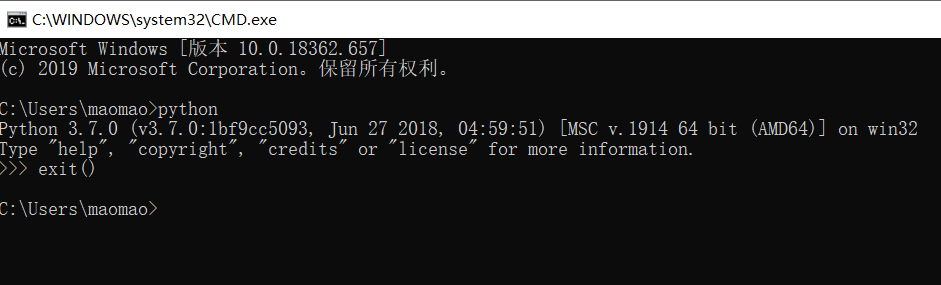2.在python各种库下载网址https://www.lfd.uci.edu/~gohlke/pythonlibs/中找到自己需要的python库。#这个网站里东西很全但找起来麻烦，建议ctrl+f查找！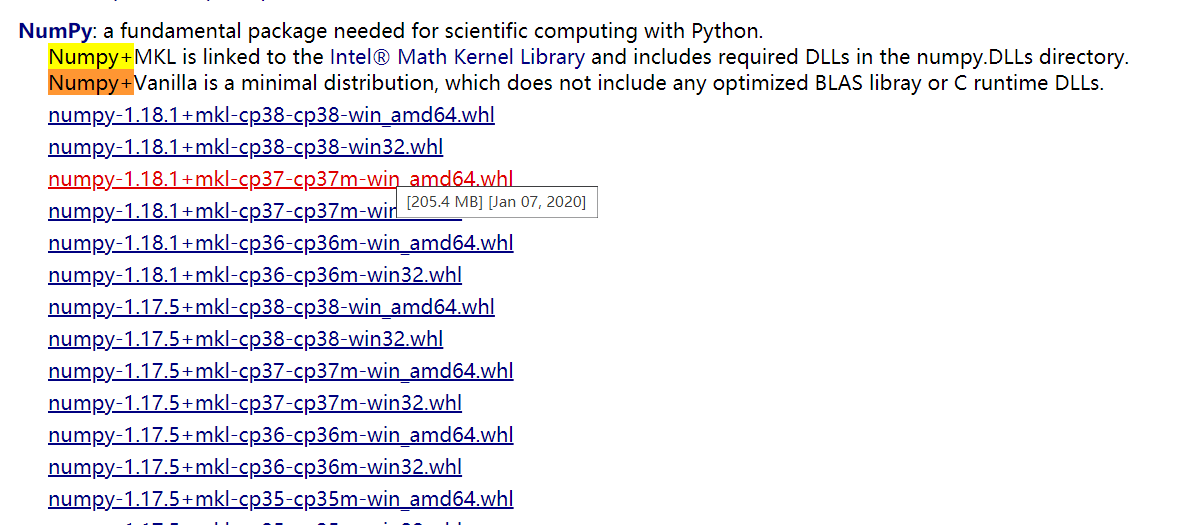3. 在1.的基础上知道自己python version，如图我是python3.7.0 以及我是windows10系统64位，我下载的numpy库就是图中标红的numpy-1.18.1+mkl-cp37-cp37m-win_amd64文件。#这个文件在上述网站下载，下载特别慢 10kb/s，我有把资源贴出来，可以去我主页下载。其他python库也按同理选择版本 4. 将下载好的库文件拷贝到自己安装python的位置，我的是D:\software-study\Python\Scripts #大家同理可得 5. 接下来在cmd命令窗口中找到自己的Scripts文件夹，如图：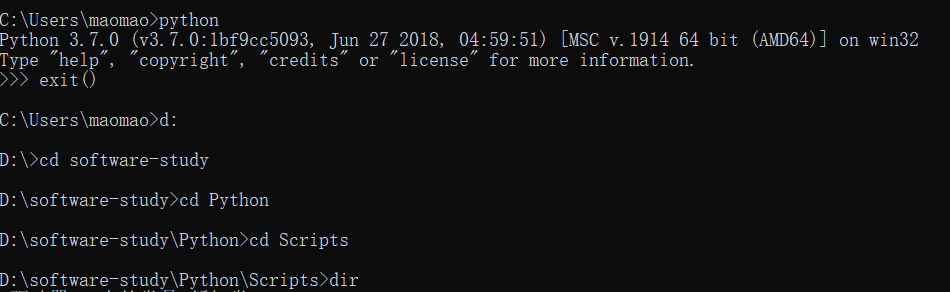#其中 d：命令 可以从c盘跳到d盘，cd 命令是打开该文件夹，dir 命令是查看该文件夹里的内容。 6. 接着用pip install命令安装你下载到Scripts文件夹里的符合版本的numpy库 如：pip install numpy-1.18.1+mkl-cp37-cp37m-win_amd64.whl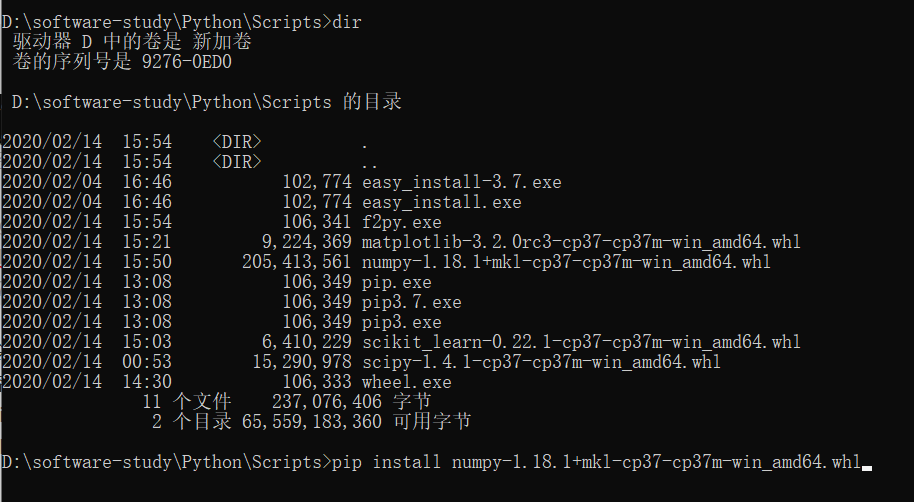#后面这一串建议通过dir命令查看文件后ctrl+c复制名称，紧接着就可以看见numpy+mkl 安装成功啦，看见successfully就可以啦。大功告成，可以通过在cmd命令行里输入python import numpy 如果不报错就成功啦！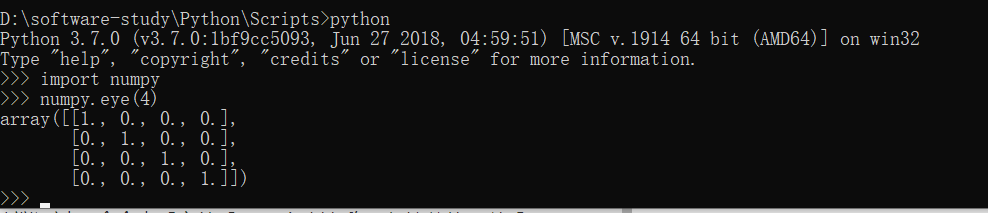import numpy numpy.eye（4） #就是输出一个4阶单位矩阵 成功啦~ 最后还可以利用pip list 命令查看自己已经安装的库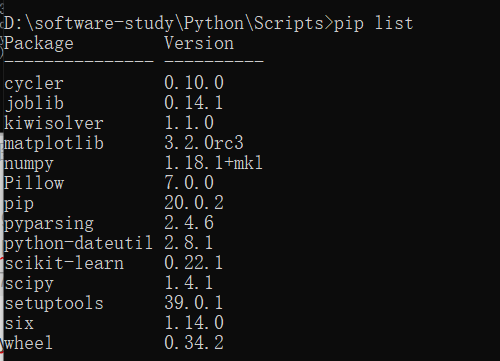7.Finally，安装scipy库、matplotlib库、sklearn库等 #同理可得， 安装命令都是在相应文件夹Scripts下输入命令 pip install （文件名）
#关于卸载 pip uninstall numpy，在y/n处输入y+回车即可卸载numpy库 卸载 pip uninstall scipy，在y/n处输入y+回车即可卸载scipy库（其他同理）
展开全文windows
• numpy安装 numpy使用说明 使用声明 np.array 数组常用属性——ndim、shape、dtype、itemsize、data 数组创建——np.zeros、np.ones、np.empty、np.ones_like、np.empty_like np.arange numpy.random.rand numpy....


numpy安装numpy使用说明

使用声明np.array数组常用属性——ndim、shape、dtype、itemsize、data数组创建——np.zeros、np.ones、np.empty、np.ones_like、np.empty_likenp.arangenumpy.random.randnumpy.random.randn通用函数numpy.dot数组索引——np改变形状——np.reshape数组叠加——np.vstack、np.hstack数组拆分——np.hsplit赋值is

NumPy系统是Python的一种开源的数值计算扩展。这种工具可用来存储和处理大型矩阵，比Python自身的嵌套列表（nested list structure)结构要高效的多（该结构也可以用来表示矩阵（matrix））。
numpy安装
在Ubuntu16.04的系统上是比较容易安装的直接执行命令
sudo pip intall numpy
需要注意的是你安装的版本是否为你需要的版本，Ubuntu 16.04中默认安装了python 3与python 2。你在python 2中安装numpy这样是不可以在python 3中使用的。
numpy使用说明
使用声明
import numpy as np
from numpy import pi
两种方式都是引入numpy库中的所有函数、函数、对象、变量等，两者的区别在于调用其中内容时不同。  以掉用numpy中的random模块为例，第一种方式要用numpy.random，第二种方式只用random即可。  特别注意：pep标准推荐使用第一种方式，请在日常使用中尽量使用第一种方法，就比如numpy中random 标准库中也有random，但是两者的功能是不同的，使用第二种方式容易造成混淆。
np.array
>>> import numpy as np
>>> a = np.array([2,3,4])
>>> a
array([2, 3, 4])
np.array为数组赋值，直接对等号右侧变量赋值array内的数组。
>>> b = np.array([(1.5,2,3), (4,5,6)])
>>> b
array([[ 1.5,  2. ,  3. ],
[ 4. ,  5. ,  6. ]])
数组常用属性——ndim、shape、dtype、itemsize、data
名称含义ndarray.ndim数组维度的数量ndarray.shape数组维度ndarray.size数组元素中的个数ndarray.dtype数组元素数据类型ndarray.itemsize每个元素所占字节数ndarray.data缓冲区包含实际的元素的数组。
示例：
>>> import numpy as np
>>> a = np.arange(15).reshape(3, 5)
>>> a
array([[ 0,  1,  2,  3,  4],
[ 5,  6,  7,  8,  9],
[10, 11, 12, 13, 14]])
>>> a.shape
(3, 5)
>>> a.ndim
2
>>> a.dtype.name
'int64'
>>> a.itemsize
8
>>> a.size
15
>>> type(a)
<type 'numpy.ndarray'>
>>> b = np.array([6, 7, 8])
>>> b
array([6, 7, 8])
>>> type(b)
<type 'numpy.ndarray'>
数组创建——np.zeros、np.ones、np.empty、np.ones_like、np.empty_like
名称含义np.zeros( (3,4) )创建3*4维0数组np.ones( (3,4) )创建3*4维1数组np.empty( (3,4) )创建3*4维空数组np.ones_like(x)创建与x维度相同的1数组np.empty_like(x)创建与x维度相同的0数组
>>> np.zeros( (3,4) )
array([[ 0.,  0.,  0.,  0.],
[ 0.,  0.,  0.,  0.],
[ 0.,  0.,  0.,  0.]])
>>> np.ones( (2,3,4), dtype=np.int16 )                # dtype can also be specified
array([[[ 1, 1, 1, 1],
[ 1, 1, 1, 1],
[ 1, 1, 1, 1]],
[[ 1, 1, 1, 1],
[ 1, 1, 1, 1],
[ 1, 1, 1, 1]]], dtype=int16)
>>> np.empty( (2,3) )                                 # uninitialized, output may vary
array([[  3.73603959e-262,   6.02658058e-154,   6.55490914e-260],
[  5.30498948e-313,   3.14673309e-307,   1.00000000e+000]])

>>> x = np.arange(6)
>>> x = x.reshape((2, 3))
>>> x
array([[0, 1, 2],
[3, 4, 5]])
>>> np.zeros_like(x)
array([[0, 0, 0],
[0, 0, 0]])
>>>
>>> y = np.arange(3, dtype=np.float)
>>> y
array([ 0.,  1.,  2.])
>>> np.zeros_like(y)
array([ 0.,  0.,  0.])

np.arange
np.arange( n, m, y )
创建一个从n开始，以y为步长增加至m的数组。
>>> np.arange( 10, 30, 5 )
array([10, 15, 20, 25])
>>> np.arange( 0, 2, 0.3 )                 # it accepts float arguments
array([ 0. ,  0.3,  0.6,  0.9,  1.2,  1.5,  1.8])
numpy.random.rand
产生固定大小元素处于（0,1）之间的随机数组
>>> np.random.rand(3,2)
array([[ 0.14022471,  0.96360618],
[ 0.37601032,  0.25528411],
[ 0.49313049,  0.94909878]])
numpy.random.randn
返回一个样本(或样品)的“标准正态分布。
>>> np.random.randn()
2.1923875335537315 #random

>>> 2.5 * np.random.randn(2, 4) + 3
array([[-4.49401501,  4.00950034, -1.81814867,  7.29718677],  #random
[ 0.39924804,  4.68456316,  4.99394529,  4.84057254]]) #random
通用函数
>>> B = np.arange(3)
>>> B
array([0, 1, 2])
>>> np.exp(B)
array([ 1.        ,  2.71828183,  7.3890561 ])
>>> np.sqrt(B)
array([ 0.        ,  1.        ,  1.41421356])
>>> C = np.array([2., -1., 4.])
array([ 2.,  0.,  6.])
这里仅仅对部分通用函数进行写出，其中还有：  all, any, apply_along_axis, argmax, argmin, argsort, average, bincount, ceil, clip, conj, corrcoef, cov, cross, cumprod, cumsum, diff, dot, floor, inner, inv, lexsort, max, maximum, mean, median, min, minimum, nonzero, outer, prod, re, round, sort, std, sum, trace, transpose, var, vdot, vectorize, where
numpy.dot
>>> a = [[1, 0], [0, 1]]
>>> b = [[4, 1], [2, 2]]
>>> np.dot(a, b)
array([[4, 1],
[2, 2]])
数组索引——np
一维数组：
>>> a = np.arange(10)**3
>>> a
array([  0,   1,   8,  27,  64, 125, 216, 343, 512, 729])
>>> a
8
>>> a[2:5]
array([ 8, 27, 64])
>>> a[:6:2] = -1000    # equivalent to a[0:6:2] = -1000; from start to position 6, exclusive, set every 2nd element to -1000
>>> a
array([-1000,     1, -1000,    27, -1000,   125,   216,   343,   512,   729])
>>> a[ : :-1]                                 # reversed a
array([  729,   512,   343,   216,   125, -1000,    27, -1000,     1, -1000])
注：**3为三次方的意思。
二维数组：
>>> b = np.fromfunction(f,(5,4),dtype=int)
>>> b
array([[ 0,  1,  2,  3],
[10, 11, 12, 13],
[20, 21, 22, 23],
[30, 31, 32, 33],
[40, 41, 42, 43]])
>>> b[2,3]
23
>>> b[0:5, 1]                       # each row in the second column of b
array([ 1, 11, 21, 31, 41])
>>> b[ : ,1]                        # equivalent to the previous example
array([ 1, 11, 21, 31, 41])
>>> b[1:3, : ]                      # each column in the second and third row of b
array([[10, 11, 12, 13],
[20, 21, 22, 23]])
改变形状——np.reshape
>>> a = np.floor(10*np.random.random((3,4)))
>>> a
array([[ 2.,  8.,  0.,  6.],
[ 4.,  5.,  1.,  1.],
[ 8.,  9.,  3.,  6.]])
>>> a.shape
(3, 4)
>>> a.reshape(6,2)  # returns the array with a modified shape
array([[ 2.,  8.],
[ 0.,  6.],
[ 4.,  5.],
[ 1.,  1.],
[ 8.,  9.],
[ 3.,  6.]])
数组叠加——np.vstack、np.hstack
>>> a = np.floor(10*np.random.random((2,2)))
>>> a
array([[ 8.,  8.],
[ 0.,  0.]])
>>> b = np.floor(10*np.random.random((2,2)))
>>> b
array([[ 1.,  8.],
[ 0.,  4.]])
>>> np.vstack((a,b))
array([[ 8.,  8.],
[ 0.,  0.],
[ 1.,  8.],
[ 0.,  4.]])
>>> np.hstack((a,b))
array([[ 8.,  8.,  1.,  8.],
[ 0.,  0.,  0.,  4.]])
数组拆分——np.hsplit
>>> a = np.floor(10*np.random.random((2,12)))
>>> a
array([[ 9.,  5.,  6.,  3.,  6.,  8.,  0.,  7.,  9.,  7.,  2.,  7.],
[ 1.,  4.,  9.,  2.,  2.,  1.,  0.,  6.,  2.,  2.,  4.,  0.]])
>>> np.hsplit(a,3)   # Split a into 3
[array([[ 9.,  5.,  6.,  3.],
[ 1.,  4.,  9.,  2.]]), array([[ 6.,  8.,  0.,  7.],
[ 2.,  1.,  0.,  6.]]), array([[ 9.,  7.,  2.,  7.],
[ 2.,  2.,  4.,  0.]])]
>>> np.hsplit(a,(3,4))   # Split a after the third and the fourth column
[array([[ 9.,  5.,  6.],
[ 1.,  4.,  9.]]), array([[ 3.],
[ 2.]]), array([[ 6.,  8.,  0.,  7.,  9.,  7.,  2.,  7.],
[ 2.,  1.,  0.,  6.,  2.,  2.,  4.,  0.]])]
赋值is
>>> a = np.arange(12)
>>> b = a            # no new object is created
>>> b is a           # a and b are two names for the same ndarray object
True
>>> b.shape = 3,4    # changes the shape of a
>>> a.shape
(3, 4)
参考：  Quickstart tutorial — NumPy v1.13.dev0 Manual
import numpy 和 from numpy import * 的区别_百度知道
展开全文• NumPy 安装Python 官网上的发行版是不包含 NumPy 模块的。我们可以使用以下几种方法来安装。1、使用已有的发行版本对于许多用户，尤其是在 Windows 上，最简单的方法是下载以下的 Python 发行版，它们包含了所有的...
• 为了画个图，被numpy这个模块的安装真的折腾疯了！！！一直装不上，花了几个小时，看了网上的很多教程、方法发现总结得不是很全，这里总结一下，防止大家再出现这个问题没有解决方法。Python的魅力之一，就是拥有...
• NumPy(Numerical Python) 是 Python 语言的一个扩展程序，支持大量的维度数组与矩阵运算，此外也针对数组运算提供大量的数学函数Python官网上的发行版是不包含NumPy模块的。（推荐学习：Python视频教程）使用 ...
• 设定份数 import numpy as np print(np.linspace(1,10,10)) print(np.linspace(1,10,num=10)) # 输出结果： # [ 1. 2. 3. 4. 5. 6. 7. 8. 9. 10.] 我们可以通过 num参数设置数据量，如果是按照参数顺序写下来，可以...人工智能
• ## python 如何安装numpy库？

万次阅读 多人点赞 2019-01-10 21:59:06
我正在参与CSDN200进20，希望得到您的支持，扫码...感谢您！ ...） 首先我们要找到python安装的位置 ...进入以后输入： where python ...找到安装目录后，找到Scripts文件夹所在位置：如Programs\Python\Python36\Scripts...
• Pythonnumpy库安装 打开cmd窗口。点击开始栏，搜索cmd并打开。 找到安装Python路径。可以通过右键点击Python快捷键，查找文件路径。（博主电脑并未分盘，故安装到了C盘，这里推荐大家安装到D盘或E盘等路径...数据挖掘
• 最近要对一系列数据做同比比较，需要用到numpy和pandas来计算，不过使用python安装numpy和pandas因为linux环境没有外网遇到了很多问题就记下来了。首要条件，python版本必须是2.7以上。linux首先安装依赖包yum -y ...
• ## Python安装numpy库

千次阅读 2019-11-21 22:42:15
1.安装python安装是否成功测试 开始-》运行->cmd： python 2.开始-》运行->cmd： pip install -i https://pypi.tuna.tsinghua.edu.cn/simple numpy 其中https://pypi.tuna.tsinghua.edu.cn/simple为清华大学...
• Python领域里有很多的与科学计算相关的包都或多或少地都使用到了Numpy这个模块包，例如著名的大数据包pandas就是基于numpy的，tensorflow的正常使用和安装也得首先安装numpy，由此可见学习numpy、学好numpy、深入...
• -------我的python3.8 ...1.Numpy库安装 点击进入之后输入pip install numpy,然后回车 安装完成之后可以输入pip show numpy检查自己是否安装成功，如果显示下面，则安装成功 2.Matplotlib库的安装 输入pyth...
• 主要为大家详细介绍了python Numpy库下载与安装图文教程，具有一定的参考价值，感兴趣的小伙伴们可以参考一下
• 安装numpy库： pip install -i https://pypi.doubanio.com/simple/ --trusted-host pypi.doubanio.com pillow （以下是废话，qaq.....，安装不成功再往下看） 昨天为了实现对一张照片灰度化处理然后成为素描化的...
• python 3.3及更高版本都内置了pip包管理器 第二步 输入 sudo pip3 install numpy（或者其他包）==版本 -i https://pypi.tuna.tsinghua.edu.cn/simple （别的我也试过不少，挺多我的都不能用） 第三步 输入密码 这里...
• python pip.exe install numpy
• python包下载网址 ...已经安装python2.7.12，查看scripts里是否有pip。2.7.9版本以上自带pip与easy_install。2.下载numpy包到python27/scripts http://www.lfd.uci.edu/~gohlke/pythonlibs/#numpy 与自己的
• ## python3.7安装Numpy库

千次阅读 2019-12-01 17:52:55
和前面的安装库语句一样，出现的问题看得是输入 pip install numpy后会发现下载的速度很慢而且到最后还不一定会安装成功，怎么解决呢？ 首先我们切盘，切到 f 盘，再找到python安装的路径（f盘是本机安装python的盘...
• NumPy是一个功能强大的Python学习，主要用于对多维数组执行计算。NumPy这个词来源于两个单词-- Numerical和PythonNumPy提供了大量的库函数和操作，可以帮助程序员轻松地进行数值计算。这类数值计算广泛用于以下...机器学习
• ## Python的Numpy库下载及安装

万次阅读 多人点赞 2019-05-17 15:57:36
** 1、首先需要下载Numpy的安装包 ** Numpy下载地址 选择跟自己系统相对应的版本，64位windows操作系统就下载这个版本 ...如果你的python版本刚好可以安装这个Numpy，那么恭喜，你的界面就会显...
• ## PythonNumPy 基础

千次阅读 2016-04-03 16:50:10
前言 这两天读完《利用Python进行数据...在往下看前请确保你已经安装了NumPy 库，并且已经使用 import numpy as np 加载numpy库。如果 还没有安装，那么可以在cmd（windows下）中使用 pip install numpy 命令安装，...数据分析
• 1.安装numpy库 NumPy库使用Python进行科学计算，尤其是数据分析时，所用到的一个基础库。它是大量Python数学和科学计算包的基础，比如我们后面会讲到的pandas库就用到了NumPy。pandas库专门用于数据分析，充分借鉴...
• 比如画图的包matplotlib需要依赖six，同时还依赖numpy、pandas等包，甚至还依赖一些。虽然使用pip管理软件，一个一个查看错误信息并按照提示安装能解决，但总是太耗费时间和精力了。   下面介绍一种很方便的...ubuntu pycharm anaconda...

# pythonnumpy库安装python 订阅